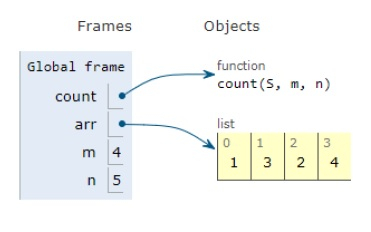# Python Program for Coin Change

Problem statement − We are given N coins and we want to make a change of those coins such that, there is an infinite supply of each value in S. we need to display that in how many ways we can make the change irrespective of order.

We will use the concept of dynamic programming to solve the problem statement to reduce the time complexity.

Now let’s observe the solution in the implementation below −

## Example

# dynamic approach
def count(S, m, n):
# base case
table = [[0 for x in range(m)] for x in range(n+1)]
# for n=0
for i in range(m):
table[i] = 1
# rest values are filled in bottom up manner
for i in range(1, n+1):
for j in range(m):
# solutions including S[j]
x = table[i - S[j]][j] if i-S[j] >= 0 else 0
# solutions excluding S[j]
y = table[i][j-1] if j >= 1 else 0
# total
table[i][j] = x + y
return table[n][m-1]
# main
arr = [1, 3, 2, 4]
m = len(arr)
n = 5
print(“Number of coins:”,end=””)
print(count(arr, m, n))

## Output

Number of coins:6All the variables are declared in the local scope and their references are seen in the figure above.

## Conclusion

In this article, we have learned about how we can make a Python Program for Coin Change